Скачать презентацию AND OR graphs Some problems are best represented

0128c2fddbd8c3393252b01c2265980c.ppt

• Количество слайдов: 21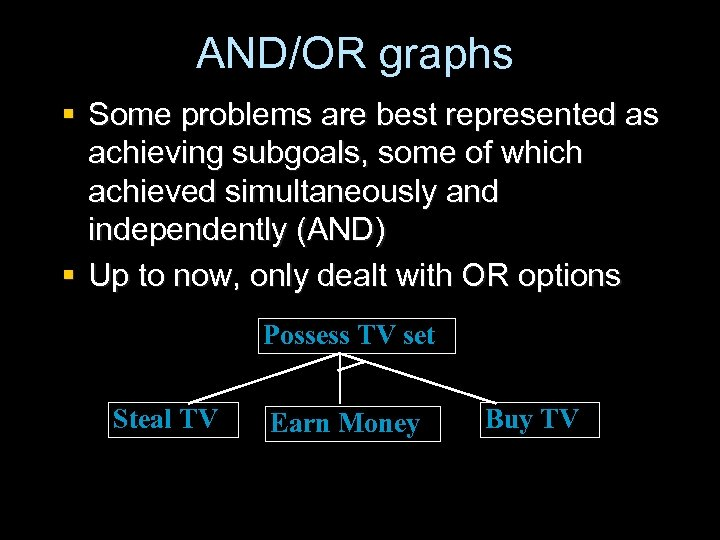AND/OR graphs § Some problems are best represented as achieving subgoals, some of which achieved simultaneously and independently (AND) § Up to now, only dealt with OR options Possess TV set Steal TV Earn Money Buy TV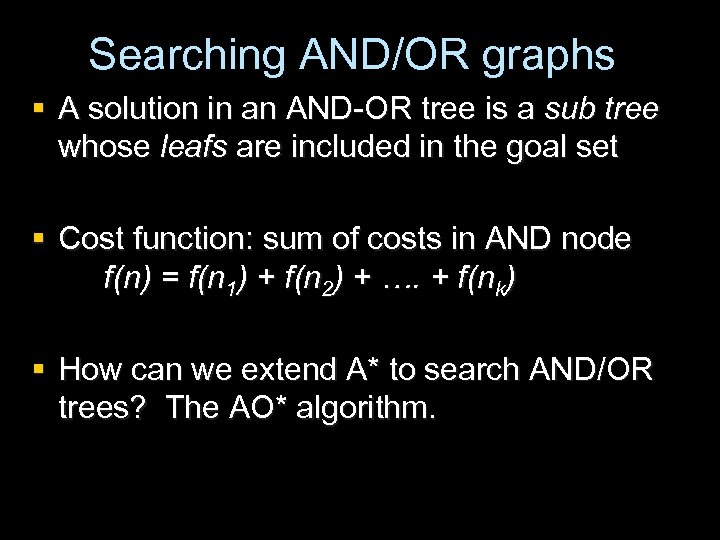Searching AND/OR graphs § A solution in an AND-OR tree is a sub tree whose leafs are included in the goal set § Cost function: sum of costs in AND node f(n) = f(n 1) + f(n 2) + …. + f(nk) § How can we extend A* to search AND/OR trees? The AO* algorithm.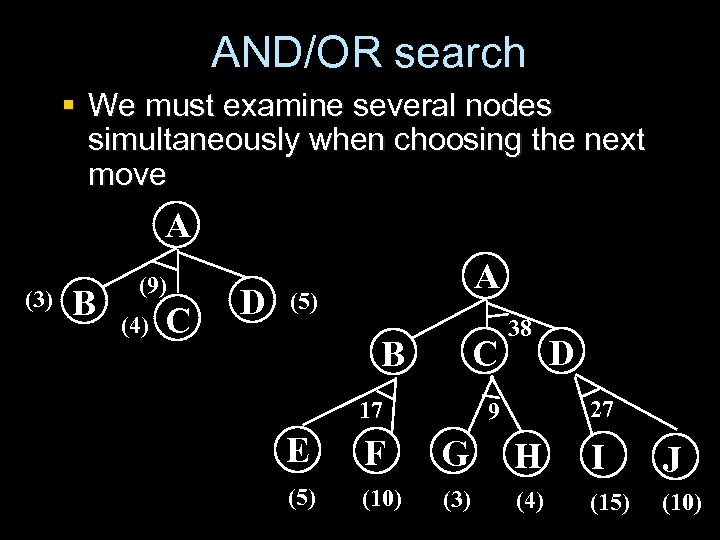AND/OR search § We must examine several nodes simultaneously when choosing the next move A (3) B (9) (4) C D A (5) C B 17 38 D 27 9 E F G H I J (5) (10) (3) (4) (15) (10)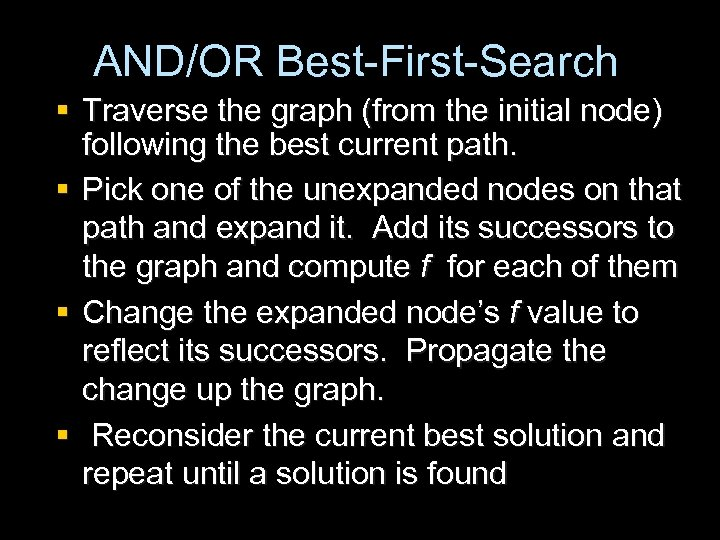AND/OR Best-First-Search § Traverse the graph (from the initial node) following the best current path. § Pick one of the unexpanded nodes on that path and expand it. Add its successors to the graph and compute f for each of them § Change the expanded node’s f value to reflect its successors. Propagate the change up the graph. § Reconsider the current best solution and repeat until a solution is found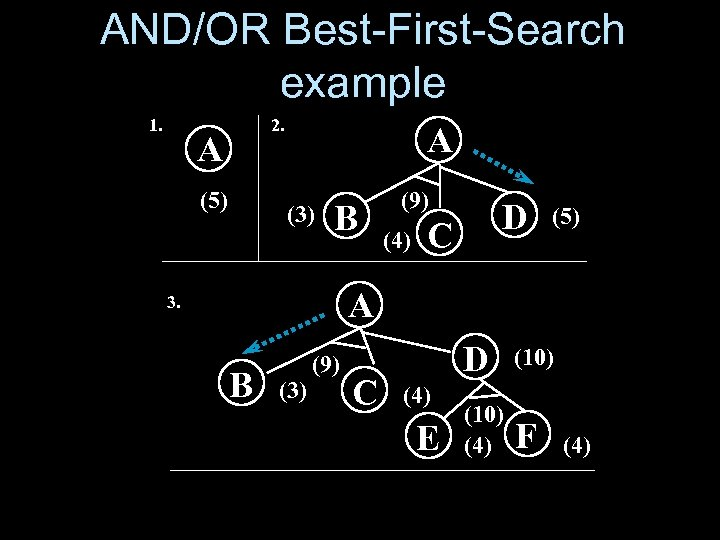AND/OR Best-First-Search example 1. 2. A (5) A (3) B (9) (4) D C (5) A 3. B (3) (9) C D (4) E (10) (4) F (4)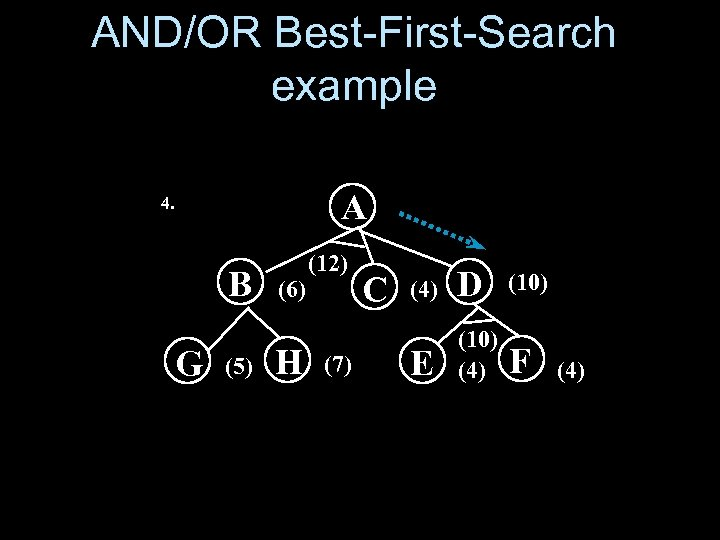AND/OR Best-First-Search example A 4. B G (5) (6) H (12) (7) C (4) D (10) E (10) (4) F (4)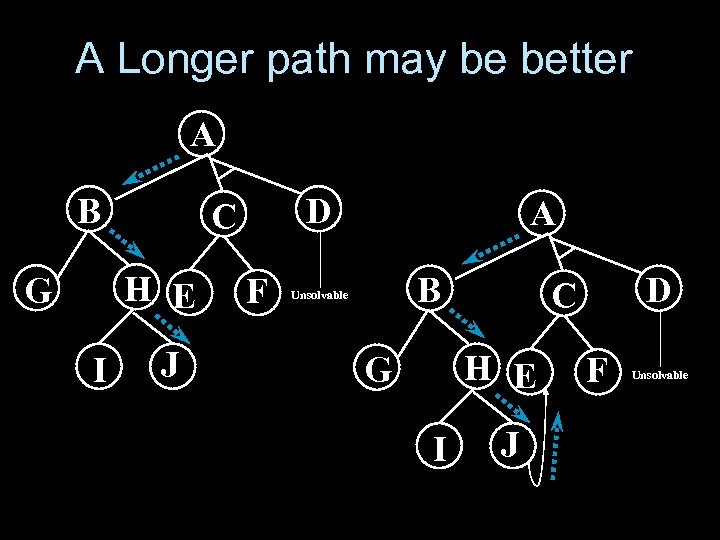A Longer path may be better A B H E G I D C J F A B Unsolvable H E G I D C J F Unsolvable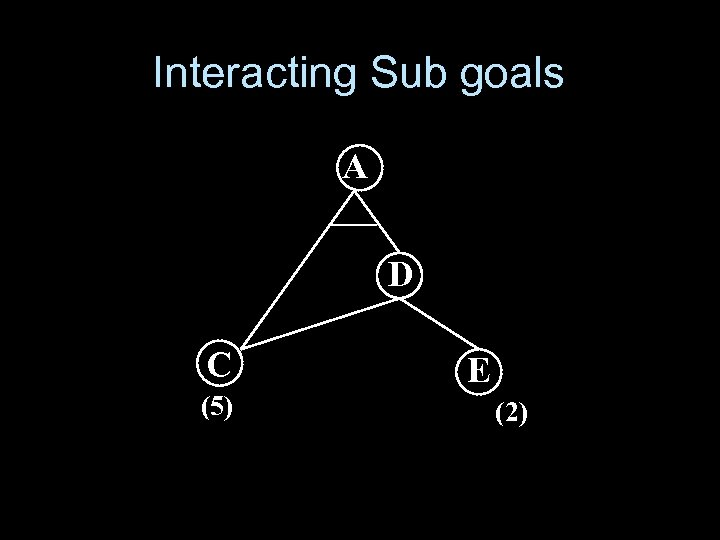Interacting Sub goals A D C (5) E (2)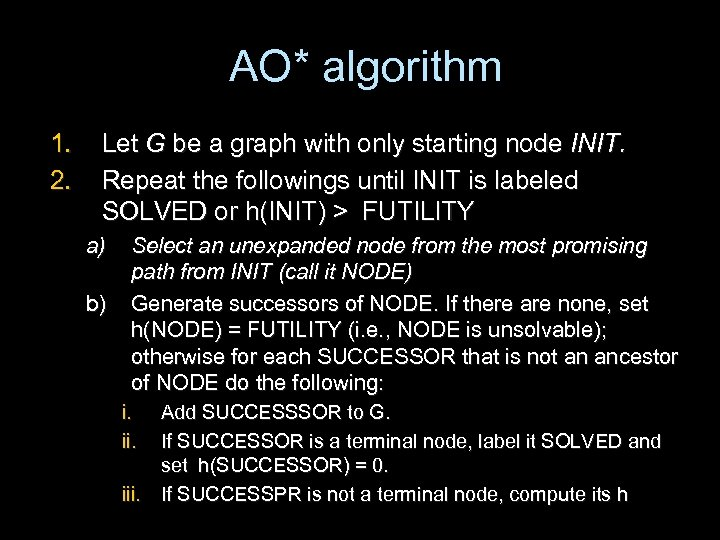AO* algorithm 1. 2. Let G be a graph with only starting node INIT. Repeat the followings until INIT is labeled SOLVED or h(INIT) > FUTILITY a) b) Select an unexpanded node from the most promising path from INIT (call it NODE) Generate successors of NODE. If there are none, set h(NODE) = FUTILITY (i. e. , NODE is unsolvable); otherwise for each SUCCESSOR that is not an ancestor of NODE do the following: i. ii. Add SUCCESSSOR to G. If SUCCESSOR is a terminal node, label it SOLVED and set h(SUCCESSOR) = 0. iii. If SUCCESSPR is not a terminal node, compute its h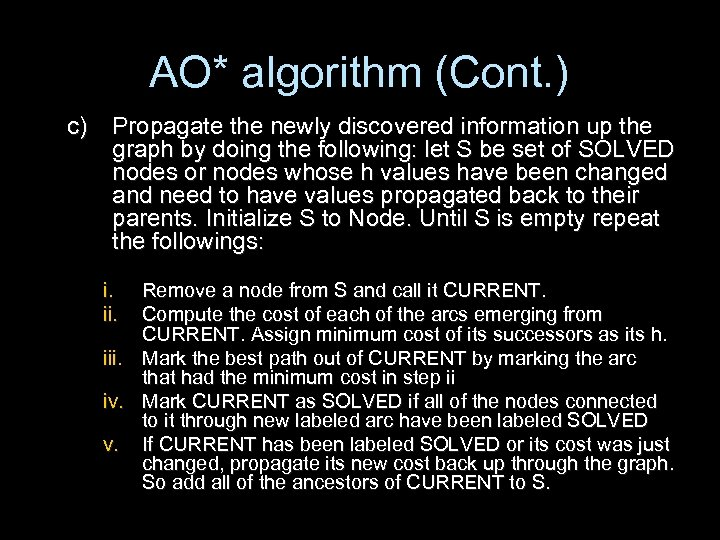AO* algorithm (Cont. ) c) Propagate the newly discovered information up the graph by doing the following: let S be set of SOLVED nodes or nodes whose h values have been changed and need to have values propagated back to their parents. Initialize S to Node. Until S is empty repeat the followings: i. ii. Remove a node from S and call it CURRENT. Compute the cost of each of the arcs emerging from CURRENT. Assign minimum cost of its successors as its h. iii. Mark the best path out of CURRENT by marking the arc that had the minimum cost in step ii iv. Mark CURRENT as SOLVED if all of the nodes connected to it through new labeled arc have been labeled SOLVED v. If CURRENT has been labeled SOLVED or its cost was just changed, propagate its new cost back up through the graph. So add all of the ancestors of CURRENT to S.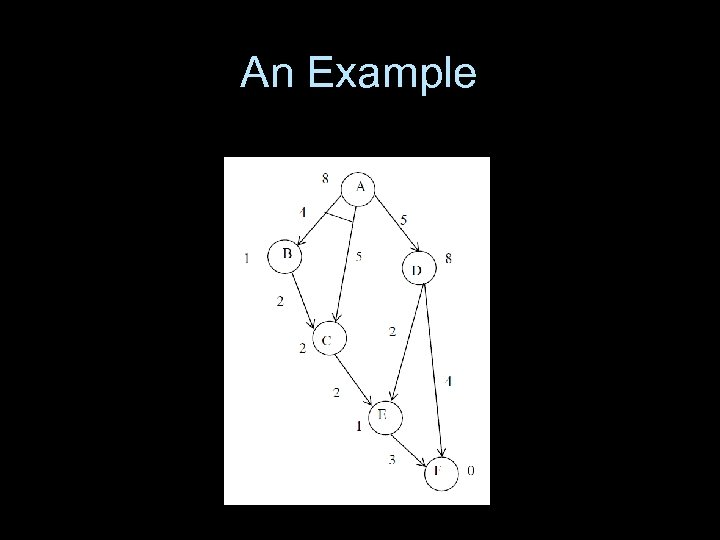An Example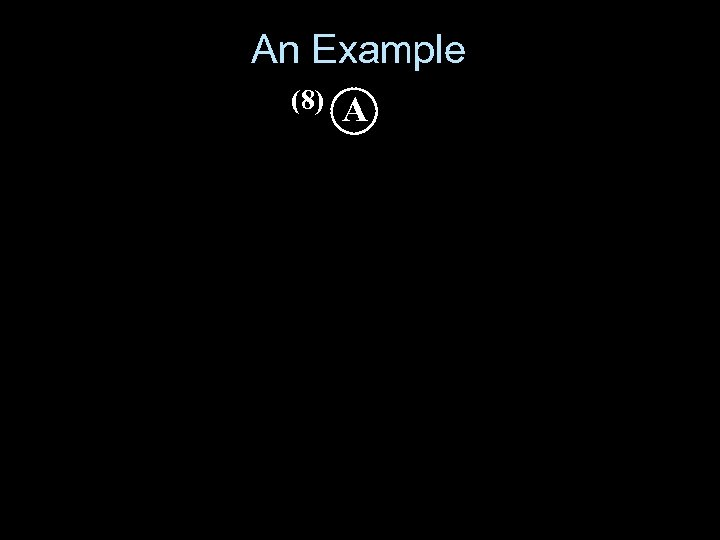An Example (8) A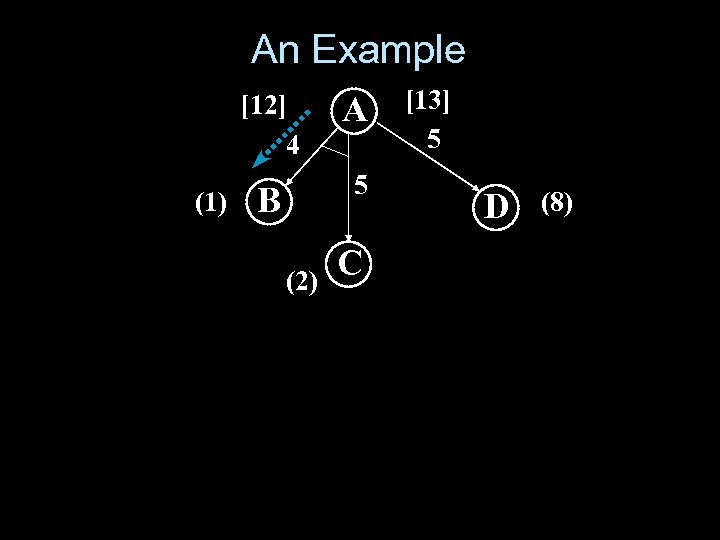An Example  4 (1) A B 5 (2) C  5 D (8)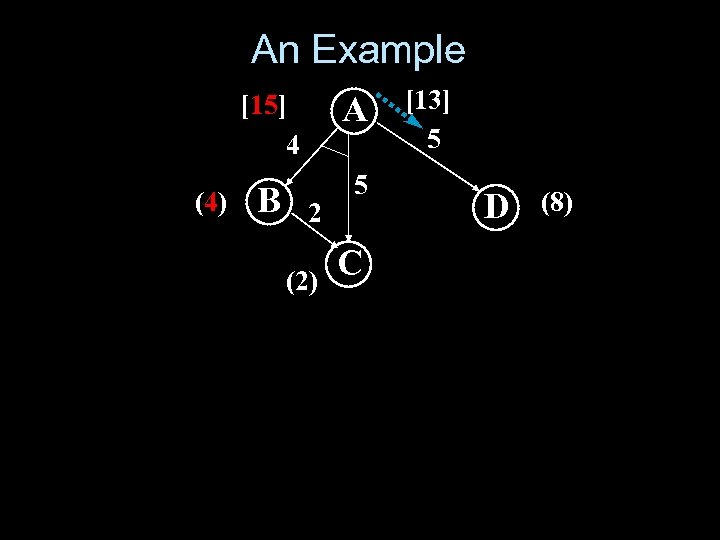An Example  4 (4) A B 5 2 (2) C  5 D (8)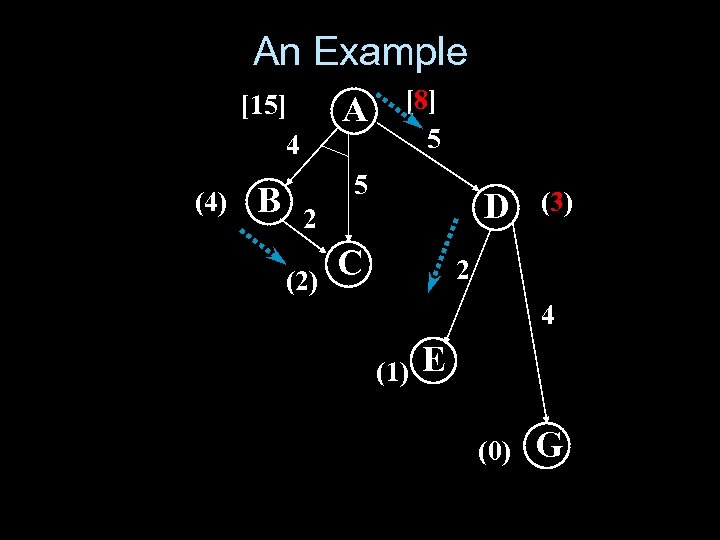An Example  4 (4) A B  5 5 D 2 (2) C (3) 2 4 (1) E (0) G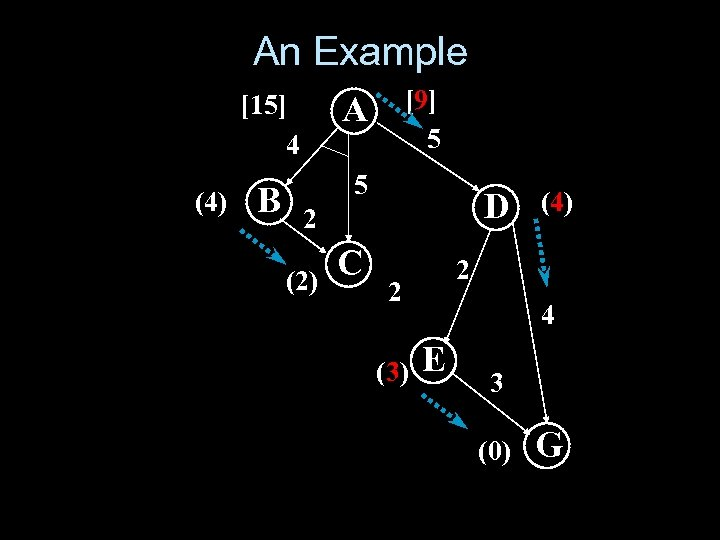An Example  4 (4) B  5 A 5 D 2 (2) C 2 2 (3) (4) 4 E 3 (0) G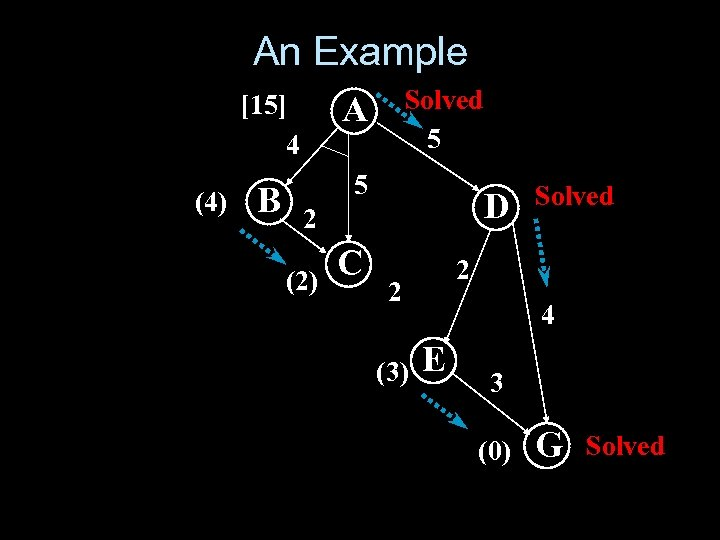An Example  4 (4) B Solved 5 A 5 D 2 (2) C 2 2 (3) Solved 4 E 3 (0) G Solved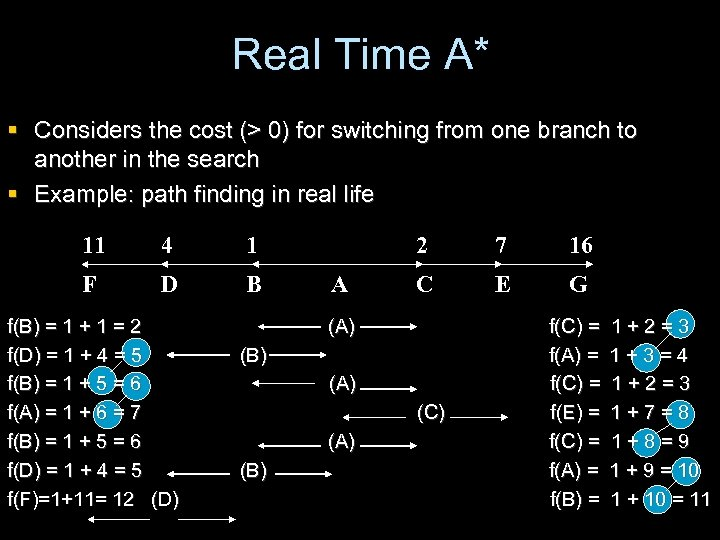Real Time A* § Considers the cost (> 0) for switching from one branch to another in the search § Example: path finding in real life 11 4 1 F D B f(B) = 1 + 1 = 2 f(D) = 1 + 4 = 5 f(B) = 1 + 5 = 6 f(A) = 1 + 6 = 7 f(B) = 1 + 5 = 6 f(D) = 1 + 4 = 5 f(F)=1+11= 12 (D) 2 A 7 16 C E G (A) (B) (A) (C) (A) (B) f(C) = f(A) = f(C) = f(E) = f(C) = f(A) = f(B) = 1+2=3 1+3=4 1+2=3 1+7=8 1+8=9 1 + 9 = 10 1 + 10 = 11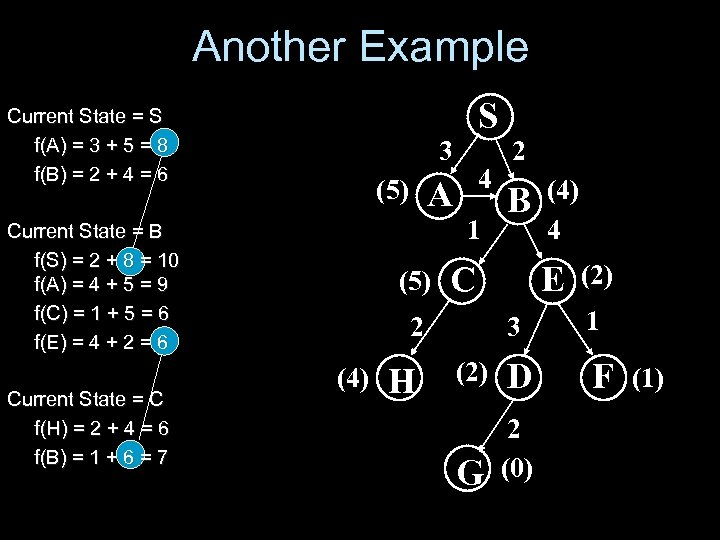Another Example S Current State = S f(A) = 3 + 5 = 8 f(B) = 2 + 4 = 6 3 1 Current State = B f(S) = 2 + 8 = 10 f(A) = 4 + 5 = 9 f(C) = 1 + 5 = 6 f(E) = 4 + 2 = 6 Current State = C f(H) = 2 + 4 = 6 f(B) = 1 + 6 = 7 4 A (5) H B C (4) 4 E (2) 3 1 (2) D F G 2 (0) 2 (4) 2 (1)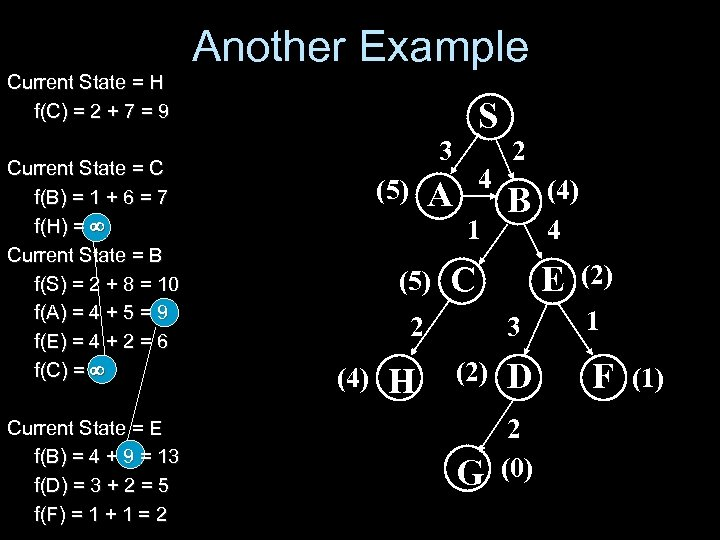Another Example Current State = H f(C) = 2 + 7 = 9 Current State = C f(B) = 1 + 6 = 7 f(H) = Current State = B f(S) = 2 + 8 = 10 f(A) = 4 + 5 = 9 f(E) = 4 + 2 = 6 f(C) = Current State = E f(B) = 4 + 9 = 13 f(D) = 3 + 2 = 5 f(F) = 1 + 1 = 2 S 3 4 A (5) 1 (5) H B C (4) 4 E (2) 3 1 (2) D F G 2 (0) 2 (4) 2 (1)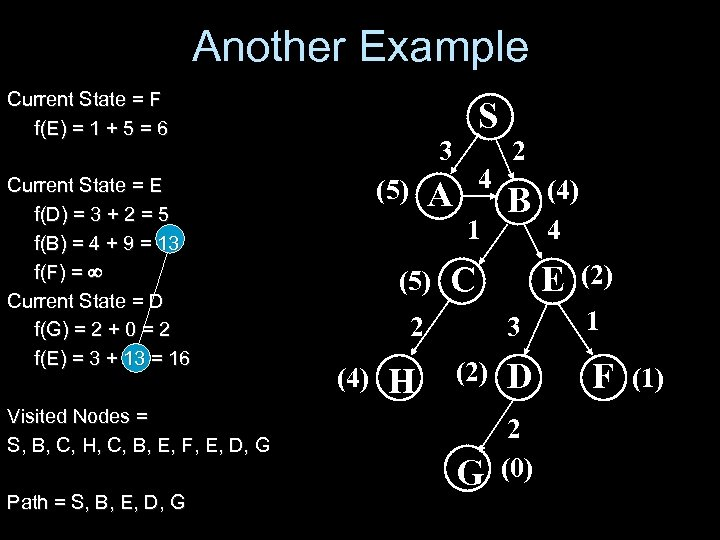Another Example Current State = F f(E) = 1 + 5 = 6 Current State = E f(D) = 3 + 2 = 5 f(B) = 4 + 9 = 13 f(F) = Current State = D f(G) = 2 + 0 = 2 f(E) = 3 + 13 = 16 Visited Nodes = S, B, C, H, C, B, E, F, E, D, G Path = S, B, E, D, G S 3 4 A (5) 1 (5) H B C (4) 4 E (2) 3 1 (2) D F G 2 (0) 2 (4) 2 (1)